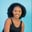Related Tags

numpy
python3
python programming

# How to use the numpy.trapz() method for 2D array in PythonMaria Elijah

## The numpy.trapz() method

The numpy.trapz() method is used to compute integration along a specified axis using the composite trapezoidal rule.

Note: A two-dimensional (2D) array can be created in Python using a list of lists.

### Syntax

numpy.trapz(y, x=None, dx=1.0, axis=- 1)


### Parameters

• y: This denotes the array of inputs to be integrated.
• x: This is array-like, but not required. It reflects the y values’ sample points. The sample points are considered to be evenly spread at a distance of dx if x is set to None. The default is None.
• dx: This is an optional scalar variable. When x is None, it reflects the distance between samples. The default value is 1.
• axis: This is the int value and optional. It represents the integration axis.

### Return value

The numpy.trapz() method returns a definite integral estimated by the trapezoidal rule. It can be a float or ndarray.

### Example

The following code shows how to use the numpy.trapz() method for two-dimensional (2D) arrays.

# import numpy
import numpy as np
# create list
x1 = [7,3,4,8]
x2 = [2,6,9,5]
# convert the lists to 2D array using np.array
y = np.array([x1,x2])

# compute the integration along a specified axis
# and store the result in result
result = np.trapz(y, dx=2)

print(result)
Using the np.trapz() function

### Explanation

• Line 2: We import the numpy library.
• Lines 4–5: We create two separate lists, x1 and x2.
• Line 7: We utilize the np.array() method to convert the lists into a 2D array. We save in a new variable called y.
• Line 11: We use np.trapz() to compute the integration along a specified axis. The result is stored in a new variable called result.
• Line 13: We display the result.

RELATED TAGS

numpy
python3
python programming

CONTRIBUTORMaria Elijah
RELATED COURSES

View all Courses

Keep Exploring

Learn in-demand tech skills in half the time